Open in App
Not now

# Interactive Data Visualization with Python and Bokeh

• Last Updated : 24 Jan, 2023

In this article, we’ll learn how to do Interactive Data Visualization with Bokeh. Bokeh is a Python library that is used for creating interactive visualizations for modern web browsers. It handles custom or specialized use cases very simply.  It provides high-performance interactive charts and plots. Bokeh output can be obtained in various mediums like a notebook, HTML, and server. It is possible to embed bokeh plots in Django and Flask apps. Here we will be exploring all the key features and code for it. So let’s get started.

To install the bokeh library run the following command in the terminal :

`pip install bokeh`
```import bokeh

### Where to use Bokeh charts?

There are various Python libraries that can be used to plot graphs and charts. If your focus is on website interactivity, then Bokeh is the better choice than other libraries. We can use the bokeh library to embed the charts on the web page, make live dashboards, etc.  Bokeh provides highly interactive graphs and plots that target modern web browsers for presentations.

### Example 1: Builtin Themes

Here, we have first imported the required libraries and then set X and Y values. Next, we’ve named the output file “output.html” A for loop is used to output all the themes available in Bokeh. Run the following code to use the built-in themes.

## Python3

 `from` `bokeh.io ``import` `curdoc``from` `bokeh.plotting ``import` `figure, output_file, show` `x ``=` `[``1``, ``2``, ``3``, ``4``, ``5``]``y ``=` `[``6``, ``7``, ``6``, ``4``, ``5``]` `output_file(``"output.html"``)``themes ``=` `[``'contrast'``, ``'night_sky'``, ``'light_minimal'``,``          ``'caliber'``, ``'dark_minimal'``]``for` `i ``in` `themes:``    ``curdoc().theme ``=` `i``    ``p ``=` `figure(title``=``i, width``=``300``, height``=``300``)``    ``p.circle(x, y)` `    ``show(p)`

Outputs: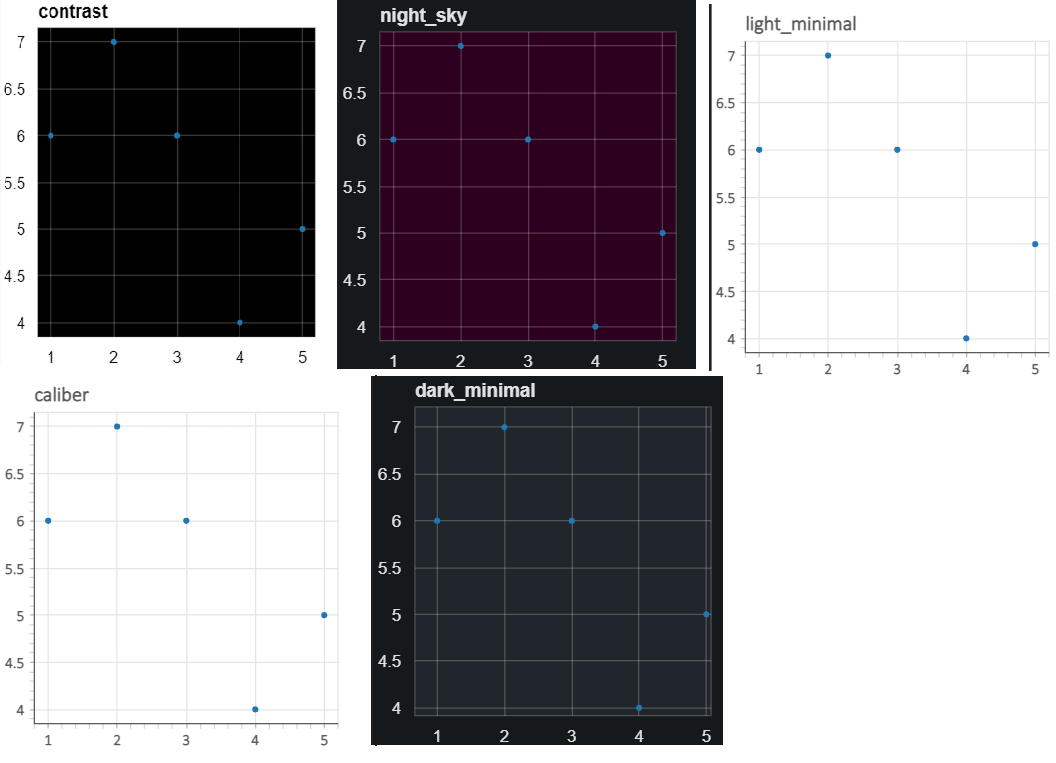### Example 2: Zoom and Drag

There are mainly 6 classifications:

• Pan/Drag Tool: The pan tool moves the graph within the plot.
• Box Zoom Tool: The box zoom tool (magnifying glass symbol) is used to zoom into an area of the plot.
• Zoom in and out Tool: In order to zoom in and out with a mouse wheel, the wheel zoom tool is used.
• Save Tool: To save the current plot as a PNG file, the save tool is used.
• Reset Tool: To reset the plot as it was before, the reset tool is used.
• Help Tool: To learn more about the tools available in Bokeh, the help tool is used.

## Python3

 `# Importing Bokeh Library``from` `bokeh.plotting ``import` `figure, output_file, show` `# Importing numpy for calculating sin values``import` `numpy as np` `x ``=` `[``1``, ``2``, ``3``, ``4``, ``5``]``y ``=` `[``6``, ``7``, ``2``, ``4``, ``5``]` `fig ``=` `figure()``fig.step(x, y, line_width``=``2``)` `# Image Showing``show(fig)`

Output:### Example 3: Configuring the Toolbar Location

By default, the position of the toolbar is above the plot. The positioning can be changed by passing  the toolbar_location parameter to the figure() function as shown below:

Syntax: Figure(*args, **kwargs)

Parameters:

• title: the title of your line chart (optional)
• width: Width of figure
• height: Height of figure.
• x_axis_label: a text label to put on the chart’s x-axis (optional)
• y_axis_label: a text label to put on the chart’s y-axis (optional)
• toolbar_location: used to set the location of the toolbar. These are “above”, “below”, “left” and “right”

In this example, we have simply added the toolbar_location parameter and set its value to “below” to set the toolbar_location below. As you can see, the toolbar is now relocated below. Similarly, you can experiment with passing other values as mentioned above.

## Python3

 `from` `bokeh.io ``import` `curdoc``from` `bokeh.plotting ``import` `figure, output_file, show` `x ``=` `[``1``, ``2``, ``3``, ``4``, ``5``]``y ``=` `[``6``, ``7``, ``6``, ``4``, ``5``]` `output_file(``"demo.html"``)`  `p ``=` `figure(title``=``'demo'``, width``=``300``, height``=``300``,``           ``toolbar_location``=``"below"``)``p.circle(x, y)` `show(p)`

Output: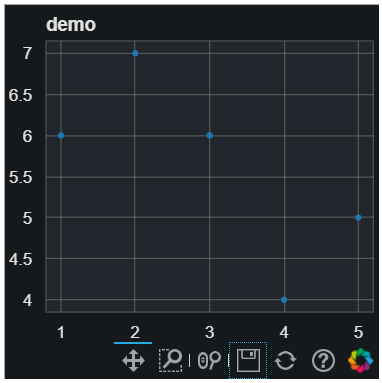### Example 4: Adding Hover Actions

The hover tool is a passive inspector tool. It is always active by default. The hover tool, by default,  generates a “tabular” tooltip where each row contains a label and its associated value. The labels and values are supplied as a list of (label, value) tuples.

TOOLTIPS = [ (“index”, “\$index”),  (“(x,y)”, “(\$x, \$y)”), (“desc”, “@desc”) ]

Note: The tooltips list consists of tuples specifying name and the field name to display.

We have defined TOOLTIPS in the above code. It is what will get displayed when we’ll hover over a data point. The labels and values are supplied as a list of (label, value) tuples. We notice that the field names begin with  \$ and @. The ones beginning with \$ are “special fields” and the field names beginning with @ are associated with columns in a ColumnDataSource.

## Python3

 `from` `bokeh.plotting ``import` `ColumnDataSource, figure, output_file, show` `output_file(``"demo.html"``)` `source ``=` `ColumnDataSource(data``=``dict``(``    ``x``=``[``1``, ``2``, ``3``, ``4``, ``5``],``    ``y``=``[``2``, ``5``, ``8``, ``2``, ``7``],``    ``desc``=``[``'A'``, ``'b'``, ``'C'``, ``'d'``, ``'E'``],``))` `TOOLTIPS ``=` `[``    ``(``"index"``, ``"\$index"``),``    ``(``"(x,y)"``, ``"(\$x, \$y)"``),``    ``(``"desc"``, ``"@desc"``),``]` `p ``=` `figure(width``=``400``, height``=``400``, tooltips``=``TOOLTIPS,``           ``title``=``"Mouse over the dots"``)` `p.circle(``'x'``, ``'y'``, size``=``20``, source``=``source)` `show(p)`

Output: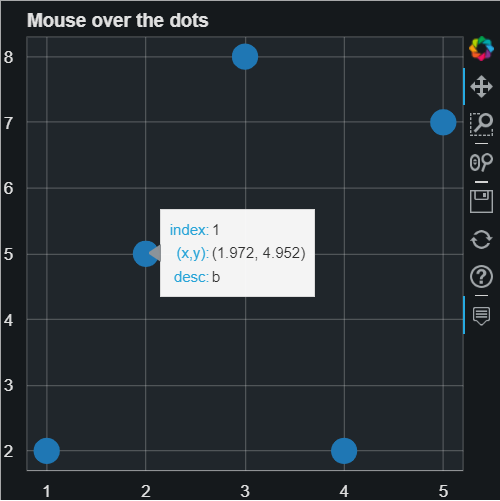### Example 5: Highlighting Data using the Legend

We have plotted a line graph with ‘Date’ as the x-axis and ‘Close’ as the y-axis. Further, we have set p.legend.click_policy to “hide” in order to hide the desirable glyph. We can hide the desirable glyph by simply clicking on an entry in a legend. Bokeh provides a feature to do so by setting the legend click_policy property to “hide” as given below:

## Python3

 `import` `pandas as pd` `from` `bokeh.palettes ``import` `Spectral4``from` `bokeh.plotting ``import` `figure, output_file, show``from` `bokeh.sampledata.stocks ``import` `AAPL, GOOG, IBM, MSFT` `p ``=` `figure(width``=``800``, height``=``250``, x_axis_type``=``"datetime"``)``p.title.text ``=` `'Click on legend entries \``            ``to hide the corresponding lines'` `for` `data, name, color ``in` `zip``([AAPL, IBM, MSFT,``                              ``GOOG], [``"AAPL"``, ``"IBM"``,``                                      ``"MSFT"``, ``"GOOG"``],``                             ``Spectral4):``    ``df ``=` `pd.DataFrame(data)``    ``df[``'date'``] ``=` `pd.to_datetime(df[``'date'``])``    ``p.line(df[``'date'``], df[``'close'``], line_width``=``2``,``           ``color``=``color, alpha``=``0.8``,``                           ``legend_label``=``name)` `p.legend.location ``=` `"top_left"``p.legend.click_policy ``=` `"hide"` `output_file(``"interactive_legend.html"``,``            ``title``=``"interactive_legend.py example"``)` `show(p)`

Output: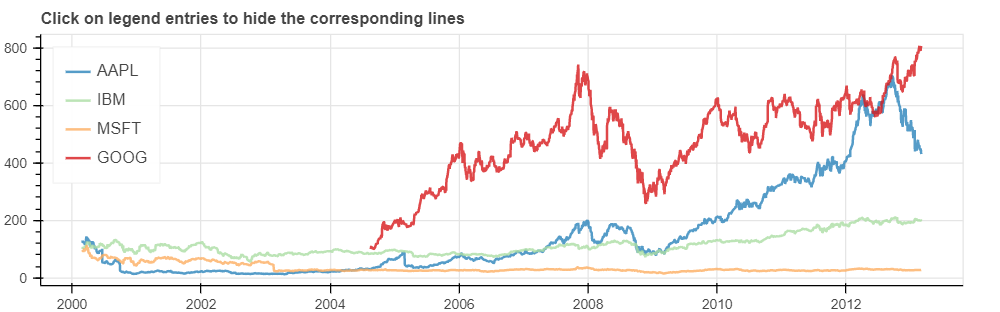### Example 6: Linking axes and selections

Linking is required when we need plots to add connected interactivity between plots. There are two types of linking:

It is used when it is required to link pan or zooming actions across more than one plot.

## Python3

 `from` `bokeh.io ``import` `output_file, show``from` `bokeh.layouts ``import` `gridplot``from` `bokeh.plotting ``import` `figure` `output_file(``"panning.html"``)` `x ``=` `list``(``range``(``11``))``y0 ``=` `x``y1 ``=` `[``10``-``xx ``for` `xx ``in` `x]``y2 ``=` `[``abs``(xx``-``5``) ``for` `xx ``in` `x]` `# create a new plot``s1 ``=` `figure(width``=``250``, height``=``250``, title``=``None``)``s1.circle(x, y0, size``=``10``, color``=``"navy"``, alpha``=``0.5``)` `# create a new plot and share both ranges``s2 ``=` `figure(width``=``250``, height``=``250``, x_range``=``s1.x_range,``            ``y_range``=``s1.y_range, title``=``None``)``s2.triangle(x, y1, size``=``10``, color``=``"firebrick"``, alpha``=``0.5``)` `# create a new plot and share only one range``s3 ``=` `figure(width``=``250``, height``=``250``, x_range``=``s1.x_range, title``=``None``)``s3.square(x, y2, size``=``10``, color``=``"olive"``, alpha``=``0.5``)` `p ``=` `gridplot([[s1, s2, s3]], toolbar_location``=``None``)` `# show the results``show(p)`

Output: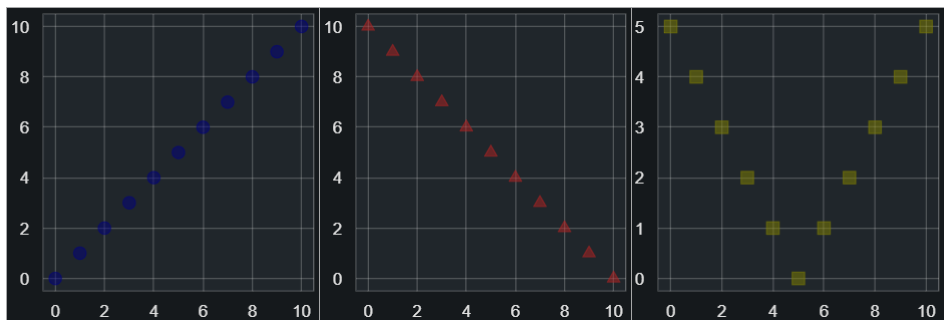Linked brushing is achieved by sharing data sources between glyph renderers.

## Python3

 `from` `bokeh.io ``import` `output_file, show``from` `bokeh.layouts ``import` `gridplot``from` `bokeh.models ``import` `ColumnDataSource``from` `bokeh.plotting ``import` `figure` `output_file(``"brushing.html"``)` `x ``=` `list``(``range``(``-``20``, ``21``))``y0 ``=` `[``abs``(xx) ``for` `xx ``in` `x]``y1 ``=` `[xx``*``*``2` `for` `xx ``in` `x]` `# create a column data source for the plots to share``source ``=` `ColumnDataSource(data``=``dict``(x``=``x, y0``=``y0, y1``=``y1))` `TOOLS ``=` `"box_select,lasso_select,help"` `# create a new plot and add a renderer``left ``=` `figure(tools``=``TOOLS, width``=``300``, height``=``300``, title``=``None``)``left.circle(``'x'``, ``'y0'``, source``=``source)` `# create another new plot and add a renderer``right ``=` `figure(tools``=``TOOLS, width``=``300``, height``=``300``, title``=``None``)``right.circle(``'x'``, ``'y1'``, source``=``source)` `p ``=` `gridplot([[left, right]])` `show(p)`

Output: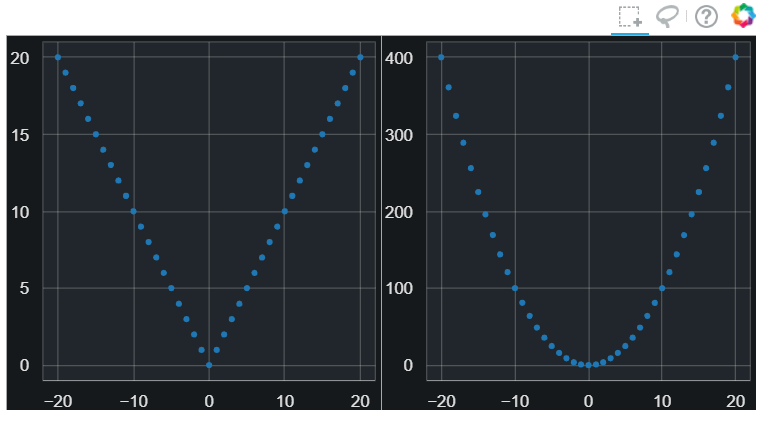### Difference between Bokeh vs Matplotlib vs Plotly

My Personal Notes arrow_drop_up